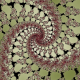This is Tupper’s Self-Referential Formula:$\displaystyle \frac12 < \left\lfloor \mathrm{mod} \left( \left\lfloor{\frac{y}{17}}\right\rfloor 2^{-17\lfloor x \rfloor - \mathrm{mod}(\lfloor y \rfloor, 17)}, 2 \right) \right\rfloor$

what makes it interesting is that the set of points$[x, y]$, for which the inequality holds, in the rectangle$0 \leq x \leq 106$,$n \leq y \leq n+16$, you will get this:Turns out that the graph of the formula resembles the formula itself, which at first sounds pretty incredible. Oh, wait: I didn’t specify the value of n. It should be equal to:

960939379918958884971672962127852754715004339660129306
651505519271702802395266424689642842174350718121267153
782770623355993237280874144307891325963941337723487857
735749823926629715517173716995165232890538221612403238
855866184013235585136048828693337902491454229288667081
096184496091705183454067827731551705405381627380967602
565625016981482083418783163849115590225610003652351370
343874461848378737238198224849863465033159410054974700
593138339226497249461751545728366702369745461014655997
933798537483143786841806593422227898388722980000748404719.

Read more about it in Wikipedia.

I spent a couple of evenings trying to figure out how does it work, and intended to write a long post about it, but I see that it has already been done here much better than I could, so I’ll only give you the main idea: the graph of the formula contains all possible combinations of pixels of size$106 \times 17$ and what you need to do is to locate the right fragment of the graph by choosing the value of n. Fortunately, the formula is constructed in such a way that it can be done rather easily. For instance, if you set n to be equal to:

770137614616740349659457383109219250729889213023196245
754997397474539522884572693040838328048207848222847384
255800207585680609541957604841348860558024608884092791
814186507612666983299083984568308963916568266347954249
445046515417964282372279838338100838479512127675315663
107944860681436794491747199511098181076928

you will get a fragment of the graph saying “hello world!”. (Oh, and it will be upside down, as pointed out in the blog post I already mentioned)

Advertisements
This entry was posted in Math. Bookmark the permalink.

### 3 Responses to

1.christopherolah says:

Very clever.

2.christopherolah says:

Someone had some fun and made a really self referential formula… http://jtra.cz/stuff/essays/math-self-reference/index.html

•George G. says:

Ah, now this is nice. Not going to try and verify it though.

(Also note that I responded to you on the “Cubed” entry.)